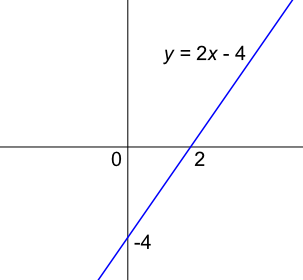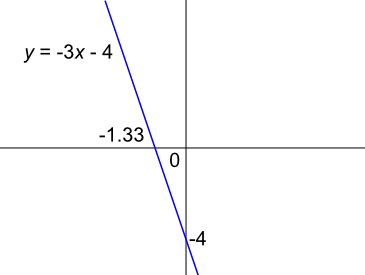Interpret Graphs of Linear Functions

# Interpret Graphs of Linear Functions

GCSE(F),

A sketch of a function will show the x and y axes and a minimum amount of data, such as where the function cross the x- and y-axes. When more than one function is plotted on the same graph, the different functions must be identified. A scale does not need to be provided:The equation of a linear function can be determined from a sketch by determining the gradient and intercept points.

## Examples

1. Sketch the function y = -3x - 4.

Answer:The -3x indicates a negative gradient, and falls from left to right. The - 4 indicates that one of the coordinates is (0, -4).

Set y to 0 and solve for x; i.e. 0 = -3x - 4 therefore x= -1.33 (2dp).

2. What are the intersection points for y = 3x + 6 on the x and y axis?

Answer: (-2, 0) and (0, 6)

Set y = 0: 0 = 3x + 6, therefore x = -2. Coordinate is (-2, 0).

Set x = 0: y = 3(0) + 6, therefore y = 6. Coordinate is (0, 6).